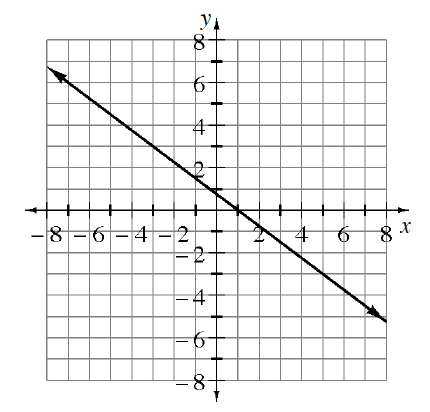### Home > CC3 > Chapter 7 > Lesson 7.2.4 > Problem7-73

7-73.

Ella and her study team are arguing about the slope of the line in the graph below. They have come up with four different answers: $\frac { 3 } { 4 }$, $- \frac { 4 } { 3 }$, $- \frac { 3 } { 4 }$ and $\frac { 4 } { 3 }$. Which slope is correct? Justify your answer.Remember that the slope can be written as $\frac{\text{change in}\ y}{\text{change in}\ x}$.

On this line as the $x$-value increases, does the $y$-value decrease or increase?
Decreasing makes the slope negative.
Increasing makes the slope positive.

Try answering this problem by going from the point $\left(−3, 3\right)$ to the point $\left(1, 0\right)$.